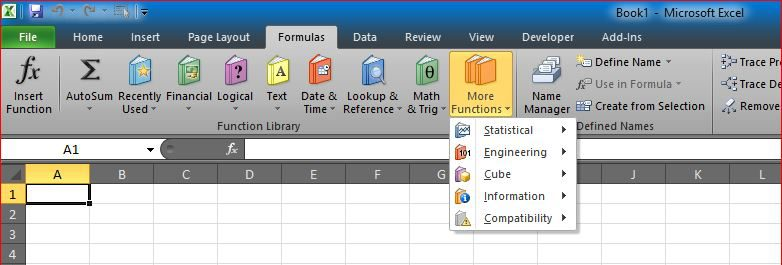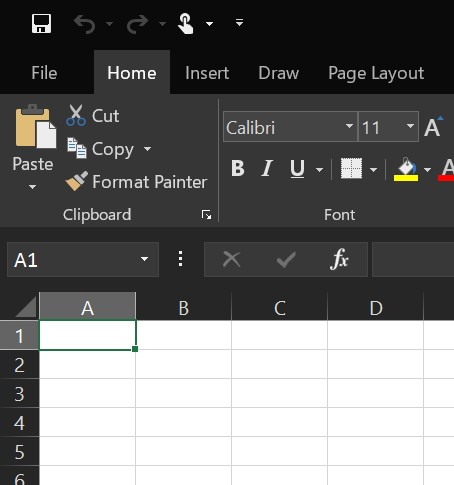# Week number to Month numberNow we need to convert week number to month number

This is exactly the same as week number to date (reverse weeknum)

So, when the week number is in A5, then formula below will get you the month number

```=IF(A5<2,MONTH(DATE(YEAR(TODAY()),1,1)),MONTH(DATE(YEAR(TODAY()),1,1)-WEEKDAY(DATE(YEAR(TODAY()),1,1))+((A5-1)*7)+1))
```

Enjoy